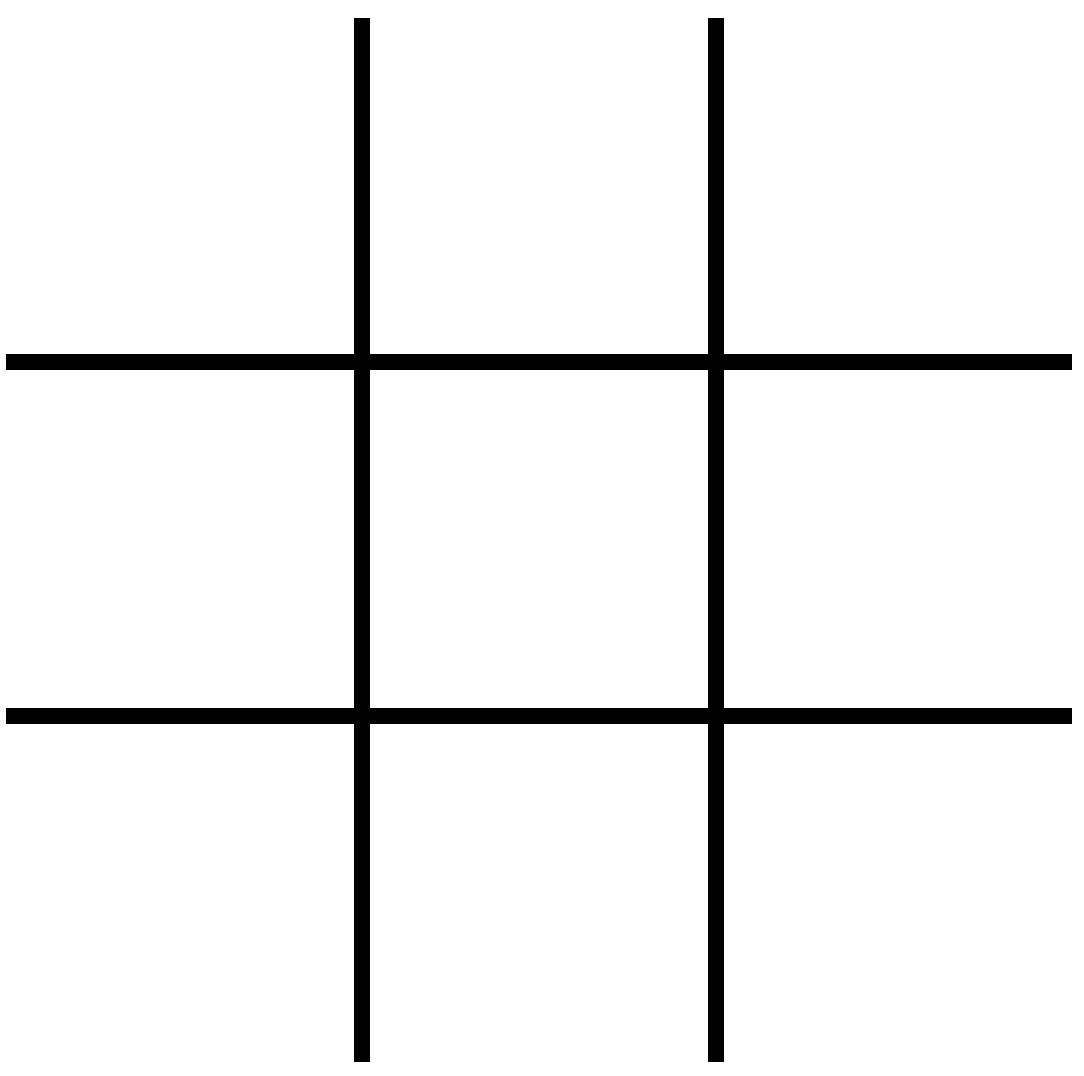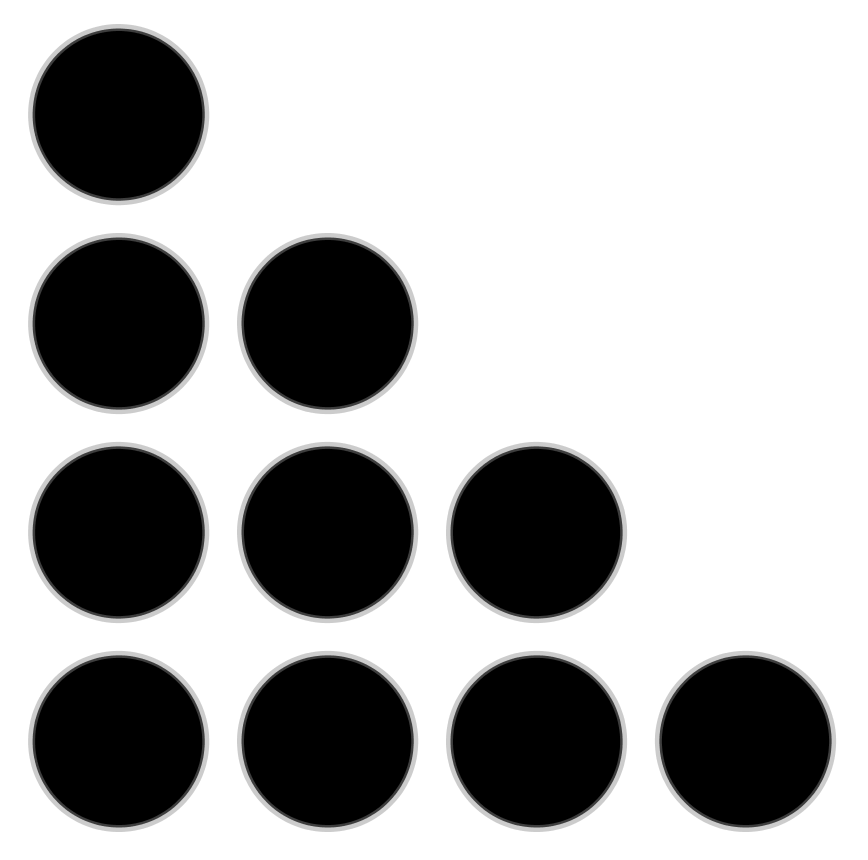# Problems to Ponder (July edition)

Welcome to this month’s edition of Problems to Ponder! Have an interesting solution? Send it to thevariable@smts.ca for publication in a future issue of The Variable!

Materials:

Directions: This game is played in pairs.

1.Place 5 counters on the ladybug.
2. Player 1 closes their eyes and Player 2 takes some of the counters off the ladybug.
3. Player 1 looks at the ladybug and determines how many counters Player 2 took off.
4. To check, players count the counters removed together.
5. Players take turns removing counters and determining how many were removed.

Extension: Use more counters as students develop proficiency with addition and subtraction.

Variation: Player 1 places counters on the ladybug. Player 2 determines how many need to be added to the ladybug to make five (says “add ____”), and places that number of counters on the ladybug.

Rectangles

Materials:

• pair of standard dice
• gameboard consisting of a 20×20 grid (p. 77 of resource)
• crayons or coloured pencils

Directions: This game is played in pairs.

1. In turn, each player rolls the dice. A player outlines and colors a rectangle on the gameboard to match the outcome. Example: a roll of 3 and 6 = a 3 x 6 rectangle or a 3 x 6 rectangle.
2. The player writes an equation to represent the total number of squares (area) in the center of the rectangle.
3. A player loses a turn when he or she rolls and cannot fit his or her rectangle on the gameboard. The game is over when neither player can draw a rectangle (alternatively, decide on a specific number of turns to be played). The winner is the player who has claimed the largest total area.

Variations/Extensions: Change the dimensions of the game board, the dice, or let each player have their own game board.

Number Tic-Tac-Toe

Draw a tic-tac-toe board (see below). Instead of using X’s and O’s, use the numbers from 1 to 9. Each number may be used only once in a game. Take turns writing a number in a space. The goal of the game is to be the first to get the numbers in any row, column, or diagonal to add up to 15.Triangle Numbers 

Ten checkers can be arranged to form a triangle, as shown in the image below.The triangle is made up of a series of lines of checkers that are placed one above the other. The longest line is at the bottom, and each of the other lines has one checker less than the line that is just below it. Because ten checkers can be arranged in a pattern like this, the number 10 is called a triangle number. If we remove the bottom line of four checkers, what’s left t is another triangle made up of six checkers, so the number 6 is also a triangle number. If we then remove the line of three checkers, what’s left is the triangle number 3. If we remove the line of two checkers, we find that the smallest triangle number is 1.

If we reverse the process, starting with 1, we can get all the triangle numbers in the order of their size. Thus, 1 is the first triangle number; 2 is the second; 6 is the third; and so on.

What is the fifth triangle number? the tenth? the hundredth? Find a shortcut (e.g., an expression) to find the nth triangle number.

Extend: Let’s call the first triangle number T(1), the second triangle number T(2), and so on. Make and complete the following list:

T(1) + T(2) =
T(2) + T(3) =
T(3) + T(4) =

What do you notice? Can you explain this pattern?

a) A car went up a hill at a speed of 90 km per hour, and returned downhill at a speed of 100 km per hour. What was the average speed for the trip?

b) A car traveled from Town A to Town B at an average speed of 50 km per hour. How fast would it have to come back to make the average speed 100 km per hour?

Sweet 16

Place the numbers from 1 to 16 in the grid below so that they obey the horizontal and vertical equations. The circles contain odd numbers and the squares contain even numbers.Sources

 Adapted from Burns, M. (1982). Math for smarty pants. Covelo, CA: Little, Brown and Company.

 Adapted from Adler, I. (1957). Magic house of numbers. New York, NY: Signet.

 Adapted from Adler, I. (1957). Magic house of numbers. New York, NY: Signet.

 Polaski, M. (2016). Sweet 16: A new numbers puzzle. Atglen, PA: Schiffer Publishing Ltd.

## 1 thought on “Problems to Ponder (July edition)”

1.Carole Hutchinson

Do anyone know if there is a vertical alignment of Sask math outcomes available from K-6?